NEET  >  NCERT Solutions: Dual Nature of Radiation & Matter - 1

# NCERT Solutions: Dual Nature of Radiation & Matter - 1 - Notes | Study Physics Class 12 - NEET

 1 Crore+ students have signed up on EduRev. Have you?

NCERT QUESTION

(Dual Nature of Radiation and Matter)

Ques 11.1:
Find the
(a) maximum frequency, and
(b) minimum wavelength of X-rays produced by 30 kV electrons.
Ans:  Potential of the electrons, V = 30 kV = 3 × 104 V
Hence, energy of the electrons, E = 3 × 104 eV
Where,
e = Charge on an electron = 1.6 × 10−19 C
(a)Maximum frequency produced by the X-rays = ν
The energy of the electrons is given by the relation:
E =
Where,
h = Planck’s constant = 6.626 × 10−34 Js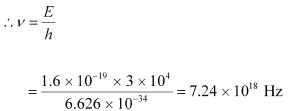Hence, the maximum frequency of X-rays produced is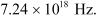(b)The minimum wavelength produced by the X-rays is given as: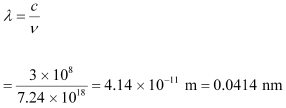Hence, the minimum wavelength of X-rays produced is 0.0414 nm.

Ques 11.2:
The work function of caesium metal is 2.14 eV. When light of frequency 6 ×1014 Hz is incident on the metal surface, photoemission of electrons occurs. What is the
(a) maximum kinetic energy of the emitted electrons,
(b) Stopping potential, and
(c) maximum speed of the emitted photoelectrons?
Ans:  Work function of caesium metal,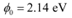Frequency of light,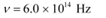(a)The maximum kinetic energy is given by the photoelectric effect as: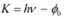Where,
h = Planck’s constant = 6.626 × 10−34 Js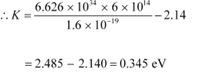Hence, the maximum kinetic energy of the emitted electrons is
0.345 eV.

(b)For stopping potential Vo , we can write the equation for kinetic energy as: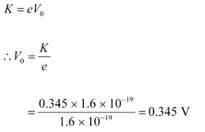Hence, the stopping potential of the material is 0.345 V.

(c) Maximum speed of the emitted photoelectrons = v

Hence, the relation for kinetic energy can be written as: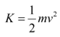Where,
m = Mass of an electron = 9.1 × 10−31 kg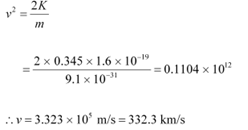Hence, the maximum speed of the emitted photoelectrons is 332.3 km/s.

Ques 11.3:
The photoelectric cut-off voltage in a certain experiment is 1.5 V. What is the maximum kinetic energy of photoelectrons emitted?
Ans:  Photoelectric cut-off voltage, V0 = 1.5 V

The maximum kinetic energy of the emitted photoelectrons is given as: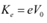Where,
e = Charge on an electron = 1.6 × 10−19 C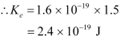Therefore, the maximum kinetic energy of the photoelectrons emitted in the given experiment is 2.4 × 10−19 J.

Ques 11.4:
Monochromatic light of wavelength 632.8 nm is produced by a helium-neon laser. The power emitted is 9.42 mW.
(a) Find the energy and momentum of each photon in the light beam,
(b) How many photons per second, on the average, arrive at a target irradiated by this beam? (Assume the beam to have uniform cross-section which is less than the target area), and
(c) How fast does a hydrogen atom have to travel in order to have the same momentum as that of the photon?
Ans:  Wavelength of the monochromatic light, λ = 632.8 nm = 632.8 × 10−9 m
Power emitted by the laser, P = 9.42 mW = 9.42 × 10−3 W
Planck’s constant, h = 6.626 × 10−34 Js
Speed of light, c = 3 × 108 m/s
Mass of a hydrogen atom, m = 1.66 × 10−27 kg

(a)The energy of each photon is given as: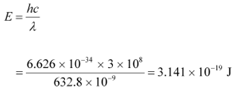The momentum of each photon is given as: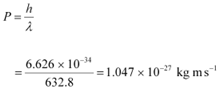(b) Number of photons arriving per second, at a target irradiated by the beam = n
Assume that the beam has a uniform cross-section that is less than the target area.
Hence, the equation for power can be written as: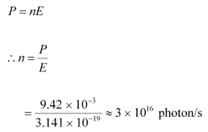(c) Momentum of the hydrogen atom is the same as the momentum of the photon,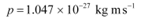Momentum is given as:

p = mv

Where,

v = Speed of the hydrogen atom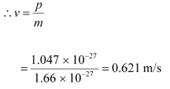Ques 11.5:
The energy flux of sunlight reaching the surface of the earth is 1.388 × 103 W/m2. How many photons (nearly) per square metre are incident on the Earth per second? Assume that the photons in the sunlight have an average wavelength of 550 nm.
Ans:  Energy flux of sunlight reaching the surface of earth, Φ = 1.388 × 103 W/m2
Hence, power of sunlight per square metre, P = 1.388 × 103 W
Speed of light, c = 3 × 108 m/s
Planck’s constant, h = 6.626 × 10−34 Js
Average wavelength of photons present in sunlight,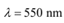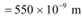Number of photons per square metre incident on earth per second = n
Hence, the equation for power can be written as: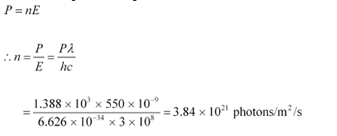Therefore, every second,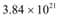photons are incident per square metre on earth.

Ques 11.6:
In an experiment on photoelectric effect, the slope of the cut-off voltage versus frequency of incident light is found to be 4.12 × 10−15 V s. Calculate the value of Planck’s constant.
Ans:  The slope of the cut-off voltage (V) versus frequency (ν) of an incident light is given as: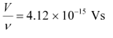v is related to frequency by the equation:
hv = ev
Where,
e = Charge on an electron = 1.6 × 10−19 C
h = Planck’s constant
e = Charge on an electron = 1.6 x 10-19 C
h = Planck`s constant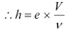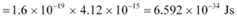Therefore, the value of Planck’s constant is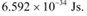Ques 11.7:
A 100 W sodium lamp radiates energy uniformly in all directions. The lamp is located at the centre of a large sphere that absorbs all the sodium light which is incident on it. The wavelength of the sodium light is 589 nm. (a) What is the energy per photon associated with the sodium light? (b) At what rate are the photons delivered to the sphere?
Ans:  Power of the sodium lamp, P = 100 W
Wavelength of the emitted sodium light, λ = 589 nm = 589 × 10−9 m
Planck’s constant, h = 6.626 × 10−34 Js
Speed of light, c = 3 × 108 m/s

(a)The energy per photon associated with the sodium light is given as: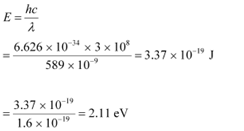(b) Number of photons delivered to the sphere = n
The equation for power can be written as: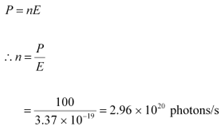Therefore, every second,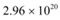photons are delivered to the sphere.

Ques 11.8:
The threshold frequency for a certain metal is 3.3 × 1014 Hz. If light of frequency 8.2 × 1014 Hz is incident on the metal, predict the cutoff voltage for the photoelectric emission.
Ans:  Threshold frequency of the metal,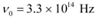Frequency of light incident on the metal,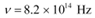Charge on an electron, e = 1.6 × 10−19 C
Planck’s constant, h = 6.626 × 10−34 Js
Cut-off voltage for the photoelectric emission from the metal = Vo
The equation for the cut-off energy is given as: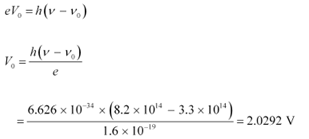Therefore, the cut-off voltage for the photoelectric emission is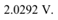Ques 11.9:
The work function for a certain metal is 4.2 eV. Will this metal give photoelectric emission for incident radiation of wavelength 330 nm?
Ans:  No
Work function of the metal,  φ0 = 4.2 eV
Charge on an electron, e = 1.6 × 10−19 C
Planck’s constant, h = 6.626 × 10−34 Js
Wavelength of the incident radiation, λ = 330 nm = 330 × 10−9 m
Speed of light, c = 3 × 108 m/s
The energy of the incident photon is given as: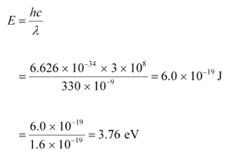It can be observed that the energy of the incident radiation is less than the work function of the metal. Hence, no photoelectric emission will take place.

Ques 11.10:
Light of frequency 7.21 × 1014 Hz is incident on a metal surface. Electrons with a maximum speed of 6.0 × 105 m/s are ejected from the surface. What is the threshold frequency for photoemission of electrons?
Ans:  Frequency of the incident photon,Maximum speed of the electrons, v = 6.0 × 105 m/s
Planck’s constant, h = 6.626 × 10−34 Js
Mass of an electron, m = 9.1 × 10−31 kg
For threshold frequency ν0, the relation for kinetic energy is written as: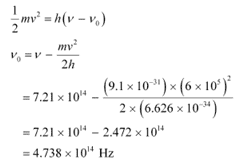Therefore, the threshold frequency for the photoemission of electrons is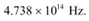Ques 11.11:
Light of wavelength 488 nm is produced by an argon laser which is used in the photoelectric effect. When light from this spectral line is incident on the emitter, the stopping (cut-off) potential of photoelectrons is 0.38 V. Find the work function of the material from which the emitter is made.
Ans:  Wavelength of light produced by the argon laser, λ = 488 nm
= 488 × 10−9 m
Stopping potential of the photoelectrons, V0 = 0.38 V
1eV = 1.6 × 10−19 J
V0 =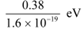Planck’s constant, h = 6.6 × 10−34 Js
Charge on an electron, e = 1.6 × 10−19 C
Speed of light, c = 3 × 10 m/s
From Einstein’s photoelectric effect, we have the relation involving the work function Φ0 of the material of the emitter as: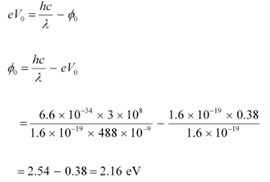Therefore, the material with which the emitter is made has the work function of 2.16 eV.

Ques 11.12:
Calculate the
(a) momentum, and
(b) de Broglie wavelength of the electrons accelerated through a potential difference of 56 V.
Ans:  Potential difference, V = 56 V
Planck’s constant, h = 6.6 × 10−34 Js
Mass of an electron, m = 9.1 × 10−31 kg
Charge on an electron, e = 1.6 × 10−19 C

(a) At equilibrium, the kinetic energy of each electron is equal to the accelerating potential, i.e., we can write the relation for velocity (v) of each electron as: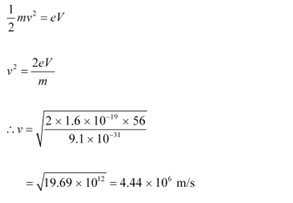The momentum of each accelerated electron is given as:
p = mv
= 9.1 × 10−31 × 4.44 × 106

= 4.04 × 10−24 kg m s−1

Therefore, the momentum of each electron is 4.04 × 10−24 kg m s−1.

(b) De Broglie wavelength of an electron accelerating through a potential V, is given by the relation: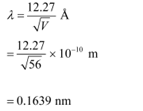Therefore, the de Broglie wavelength of each electron is 0.1639 nm.

Ques 11.13:
What is the
(a) momentum,
(b) speed, and
(c) de Broglie wavelength of an electron with kinetic energy of 120 eV.
Ans:  Kinetic energy of the electron, Ek = 120 eV
Planck’s constant, h = 6.6 × 10−34 Js
Mass of an electron, m = 9.1 × 10−31 kg
Charge on an electron, e = 1.6 × 10−19 C
(a) For the electron, we can write the relation for kinetic energy as: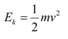Where,
v = Speed of the electron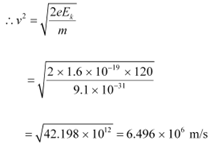Momentum of the electron, p = mv
= 9.1 × 10−31 × 6.496 × 106
= 5.91 × 10−24 kg m s−1

Therefore, the momentum of the electron is 5.91 × 10−24 kg m s−1.

(b) Speed of the electron, v = 6.496 × 106 m/s
(c) De Broglie wavelength of an electron having a momentum p, is given as: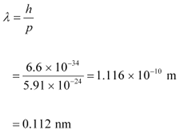Therefore, the de Broglie wavelength of the electron is 0.112 nm.

Ques 11.14:
The wavelength of light from the spectral emission line of sodium is 589 nm. Find the kinetic energy at which
(a) an electron, and
(b) a neutron, would have the same de Broglie wavelength.
Ans:  Wavelength of light of a sodium line, λ = 589 nm = 589 × 10−9 m
Mass of an electron, me= 9.1 × 10−31 kg
Mass of a neutron, mn= 1.66 × 10−27 kg
Planck’s constant, h = 6.6 × 10−34 Js

(a) For the kinetic energy K, of an electron accelerating with a velocity v, we have the relation: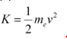We have the relation for de Broglie wavelength as: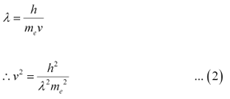Substituting equation (2) in equation (1), we get the relation: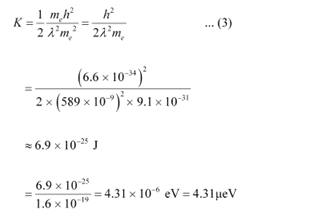Hence, the kinetic energy of the electron is 6.9 × 10−25 J or 4.31 μeV.

(b) Using equation (3), we can write the relation for the kinetic energy of the neutron as: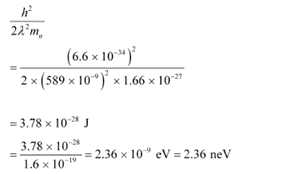Hence, the kinetic energy of the neutron is 3.78 × 10−28 J or 2.36 neV.

Ques 11.15:
What is the de Broglie wavelength of
(a) a bullet of mass 0.040 kg travelling at the speed of 1.0 km/s,
(b) a ball of mass 0.060 kg moving at a speed of 1.0 m/s, and
(c) a dust particle of mass 1.0 × 10−9 kg drifting with a speed of 2.2 m/s?
Ans:  (a)Mass of the bullet, m = 0.040 kg
Speed of the bullet, v = 1.0 km/s = 1000 m/s
Planck’s constant, h = 6.6 × 10−34 Js
De Broglie wavelength of the bullet is given by the relation: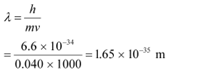(b) Mass of the ball, m = 0.060 kg
Speed of the ball, v = 1.0 m/s
De Broglie wavelength of the ball is given by the relation: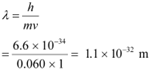(c) Mass of the dust particle, m = 1 × 10−9 kg
Speed of the dust particle, v = 2.2 m/s
De Broglie wavelength of the dust particle is given by the relation: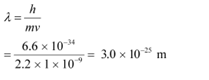Ques 11.16:
An electron and a photon each have a wavelength of 1.00 nm. Find
(a) their momenta,
(b) the energy of the photon, and
(c) the kinetic energy of electron.
Ans:  Wavelength of an electron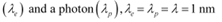= 1 × 10−9 m

Planck’s constant, h = 6.63 × 10−34 Js

(a) The momentum of an elementary particle is given by de Broglie relation: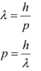It is clear that momentum depends only on the wavelength of the particle. Since the wavelengths of an electron and a photon are equal, both have an equal momentum.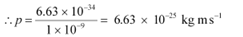(b) The energy of a photon is given by the relation: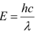Where,
Speed of light, c = 3 × 108 m/s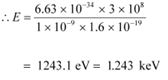Therefore, the energy of the photon is 1.243 keV.

(c) The kinetic energy (K) of an electron having momentum p,is given by the relation: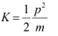Where,
m = Mass of the electron = 9.1 × 10−31 kg
p = 6.63 × 10−25 kg m s−1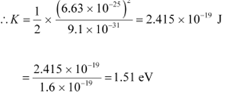Hence, the kinetic energy of the electron is 1.51 eV.

Ques 11.17:
(a) For what kinetic energy of a neutron will the associated de Broglie wavelength be 1.40 × 10−10 m?
(b) Also find the de Broglie wavelength of a neutron, in thermal equilibrium with matter, having an average kinetic energy of (3/2) kT at 300 K.
Ans:  (a) De Broglie wavelength of the neutron, λ = 1.40 × 10−10 m
Mass of a neutron, mn = 1.66 × 10−27 kg
Planck’s constant, h = 6.6 × 10−34 Js
Kinetic energy (K) and velocity (v) are related as: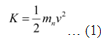De Broglie wavelength (λ) and velocity (v) are related as: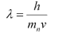..... (2)

Using equation (2) in equation (1), we get: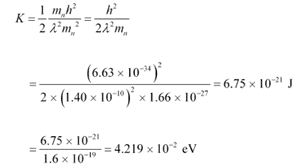Hence, the kinetic energy of the neutron is 6.75 × 10−21 J or 4.219 × 10−2 eV.

(b) Temperature of the neutron, T = 300 K
Boltzmann constant, k = 1.38 × 10−23 kg m2 s−2 K−1
Average kinetic energy of the neutron: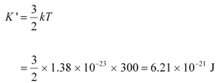The relation for the de Broglie wavelength is given as: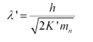where,
mn = 1.66 x 10-27 kg
h = 6.6 x 10-34 Js
K` = 6.75 x 10-21 J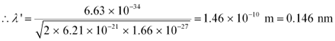Therefore, the de Broglie wavelength of the neutron is 0.146 nm.

Ques 11.18:
Show that the wavelength of electromagnetic radiation is equal to the de Broglie wavelength of its quantum (photon).
Ans:  The momentum of a photon having energy () is given as: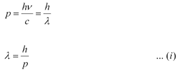Where,
λ = Wavelength of the electromagnetic radiation
c = Speed of light
h = Planck’s constant
De Broglie wavelength of the photon is given as: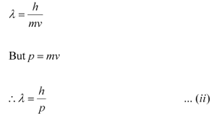Where,
m = Mass of the photon
v = Velocity of the photon
Hence, it can be inferred from equations (i) and (ii) that the wavelength of the electromagnetic radiation is equal to the de Broglie wavelength of the photon.

The document NCERT Solutions: Dual Nature of Radiation & Matter - 1 - Notes | Study Physics Class 12 - NEET is a part of the NEET Course Physics Class 12.
All you need of NEET at this link: NEET

## Physics Class 12

157 videos|452 docs|213 tests
 Use Code STAYHOME200 and get INR 200 additional OFF

## Physics Class 12

157 videos|452 docs|213 tests

### How to Prepare for NEET

Read our guide to prepare for NEET which is created by Toppers & the best Teachers

Track your progress, build streaks, highlight & save important lessons and more!

,

,

,

,

,

,

,

,

,

,

,

,

,

,

,

,

,

,

,

,

,

;